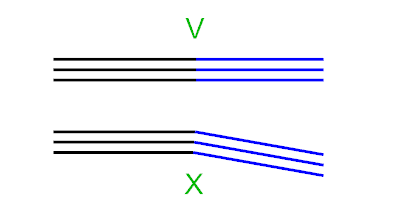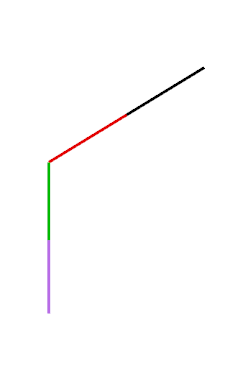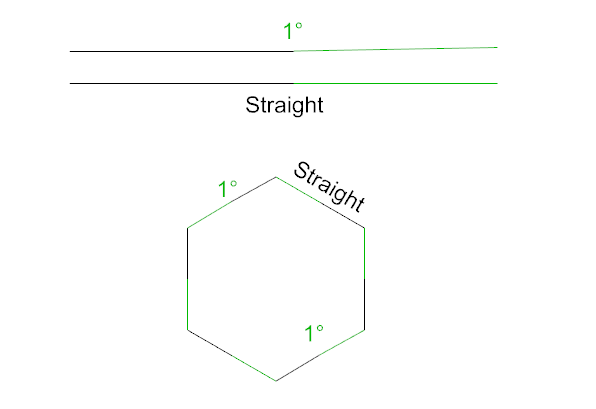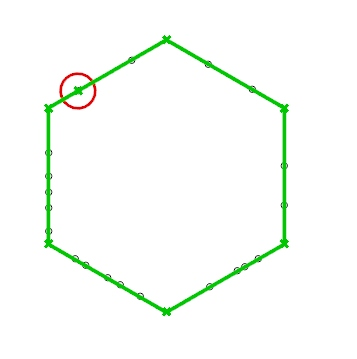# Best way to test if two curves are tangent?

Hi friends,

I have these two straight lines and I’d like to know if they are tangent (180°) with each other:

What would be the best way to test this in GH?

I’ve tried checking the angular dimension between the curves but the component doesn’t output anything so it could tell me it’s 180 degrees between them but I can’t use the 180 value, just view it…
It could be beneficial for me to get the angle value so I could work with it… is there a better way to do this?

Roy.

Stating the obvious: grasshopper angles are in radians.
Pi = 180° = 3.14159

Line objects (which in grasshopper are a particular case of curve), can be directly converted into vectors.

You can use the Vector Angle component to measure the angle, and when the two of them are tangent you should expect a zero-ish value. You can make a test if the value is under a tolerance like 0.001 you say they are tangent.

Note: the 2 lines (converted to vector) create a 0 angle when their direction is the same, like both pointing towards the right of the picture.
A 180° or better Pi angle would mean Line A direction is to the left, and B to the right, or viceversa.

1 Like

Thanks a lot Riccardo.

I got the angle which got me half way in my quest to join the curves if tangent…

Still missing a condition methodI have a lot of lines and I’m trying to “Join all the straight continuous lines only”You can join them than use condition if the joined curves IsLinear

1 Like

Thanks for you help @seghierkhaled

I can’t join them before hand since some of them looks like this:I need the purple and green to join and the red and black to join,

But I don’t want the green and red to join… that’s why I was thinking to test it by the angle…

Thanks again.

Post a file with all cases, this better than posting a new problem everytime

1 Like
1 Like

I couldn’t expect that you’ll suggest to join everything in advance as I was trying to find a solution using the angle, so I only though about this case after your suggestion. Those are all the cases…

Thanks a lot, I will try that.

Thank you @seghierkhaled , It worked and it’s a good solution, But I was just thinking that I wouldn’t be able to have any tolerance using this method, it would be dead straight or it won’t join… I’m sorry as I’m thinking about it as I goThat is why I was more after comparing the angle of any two lines, maybe sometimes there will be a up to 1 degree angle between the lines or so and that should still join…

That’s why posting a file is better, this help people to help you and give you many solutions

You are right that’s why I’m posting images, I don’t have a file as meanwhile it’s theoretical, I could have any lines in the future.There could be up to 1 degree angle between some straight lines. again that’s why I was looking for the angle before you’re solution.

I need to join all the curves that are straight or almost straight with each other (179° or more and 181° or less).

Many Thanks!

But how would you be sure that your curves are in the correct order?.
Without the correct order how is it possible to compare a angle?.
Splitting the curve at a specific value later is a better way to go.
You could use the same logic from this thread but instead of culling just split at the false and not the true member

You see, again a new condition.
You know to find solutions we must draw many cases from your drawings and explanations than you create a new condition and we create new lines, why you don’t draw your lines with all your conditions and share the file?

2 Likes

An apparent solution is to use the `Vector3d.IsParallelTo` method in a script:

220716_LinesParallel_GHPython_00.gh (9.2 KB)

I was taking a little time to learn and explore your suggestions.

@seghierkhaled @maje90 Thanks for your help, again, I didn’t think of the later case when I asked the question so it was impossible for me to ask you about it.

@seghierkhaled Thanks for your solution and your effort, it worked but I had a little issue that one segment of a line still had a point:This polyline is built of many small segments that ends where the red points are,
In the red circle you can see a left over segment, changing the tolerance didn’t help to change it.

@flokart Thanks for your solution! It works great, it is similar to @seghierkhaled’s and it solves this as well…

It also had the issue as in the image above but I’ve managed to fix it by changing the tolerance to anything which is equal or above 0.001 (degrees). So it works and that is great.

@AndersDeleuran Thanks for your solution as well, it does answer my original question but I didn’t think of another case that might happen only by later on.

Thanks a lot everyone again for your help!

Roy.

A thought: do you want to join two lines with a kink in the middle, or do you actually want to replace the two lines with a single straight line?

The most obvious solution wasn’t mentioned here at all (or I missed it).

“Best way to test if two curves are tangent?”

Foremost, some clarification on terms. A line is a subtype of a curve. Actually, it’s a curve with no curvature. But a line is not equal to a curve!
In my opinion, the best way is when you understand what’s going on. Sure you can make library calls all day, but if you want to learn something then you might want to understand the math underneath…

The tangent of a line is

`vTan = pEnd - pStart. `

The length of the vector we get (which is the same as the length of the line)

`length = Sqrt(x²+y²+z²) `

By dividing the vTan with its length, we get its normalized vector (length = 1)

`|vTan| = vTan / length`

Now, when having two lines, we know if they are parallel if the dot-product of both normalized tangents are 1. They are antiparallel if the dot is -1.

To compare two floating numbers, you usually do an epsilon equal comparison. In its simplest form
you compare two number within a given tolerance, by saying if the abs(b-a) < tolerance.

By taking the arccos(dot) you get the angles in rad. Just in case you want to epsilon equal in angles.

Of course you also need to check if line A and line B are on the same line. Therefore you need to compute a vector by picking two points on each line. By checking if the vector is again parallel or antiparallel to the tangent you know if lineA is linear. As alternative you can check if one endpoint is positional aligned to another endpoint on the other curve. Then you basically check for positional continuity. (So you epsilon equal two end-points.)

(On a NurbsCurve this is a bit more complex, because the tangent is the first derivative of the parametric equation at a given parameter. But essentially, you don’t need to do this math at all. Because Grasshopper offers you some components to get the Tangent using the EvalCurve component. By extracting the tangent, of a curve, you also get the normalized vector. So you only need to compute the dot and make the comparison)

1 Like